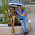## lunedì 7 maggio 2007

### Make many barplot into one plot

```# I have 4 tables like this:
satu <- array(c(5,15,20,68,29,54,84,119), dim=c(2,4), dimnames=list(c("Negative", "Positive"), c("Black", "Brown", "Red", "Blond")))
dua <- array(c(50,105,30,8,29,25,84,9), dim=c(2,4), dimnames=list(c("Negative", "Positive"), c("Black", "Brown", "Red", "Blond")))
tiga <- array(c(9,16,26,68,12,4,84,12), dim=c(2,4), dimnames=list(c("Negative", "Positive"), c("Black", "Brown", "Red", "Blond")))
empat <- array(c(25,13,50,78,19,34,84,101), dim=c(2,4), dimnames=list(c("Negative", "Positive"), c("Black", "Brown", "Red", "Blond")))
# rbind() the tables together
TAB <- rbind(satu, dua, tiga, empat)
# Do the barplot and save the bar midpoints
mp <- barplot(TAB, beside = TRUE, axisnames = FALSE)
# Add the individual bar labels
mtext(1, at = mp, text = c("N", "P"),
line = 0, cex = 0.5)
# Get the midpoints of each sequential pair of bars
# within each of the four groups
at <- t(sapply(seq(1, nrow(TAB), by = 2),
function(x) colMeans(mp[c(x, x+1), ])))
# Add the group labels for each pair
mtext(1, at = at, text = rep(c("satu", "dua", "tiga", "empat"), 4),
line = 1, cex = 0.75)
# Add the color labels for each group
mtext(1, at = colMeans(mp), text = c("Black", "Brown", "Red", "Blond"), line = 2)```
``

#### 3 commenti:

1.Thank you this is very useful! Do you know if you could do the same with tables of different dimensions?
I tried to create a barplot with tables of different numbers of rows. I end up with gaps where there are NAs

2.thank you so much for the help

3.I'm glad it was of some help!